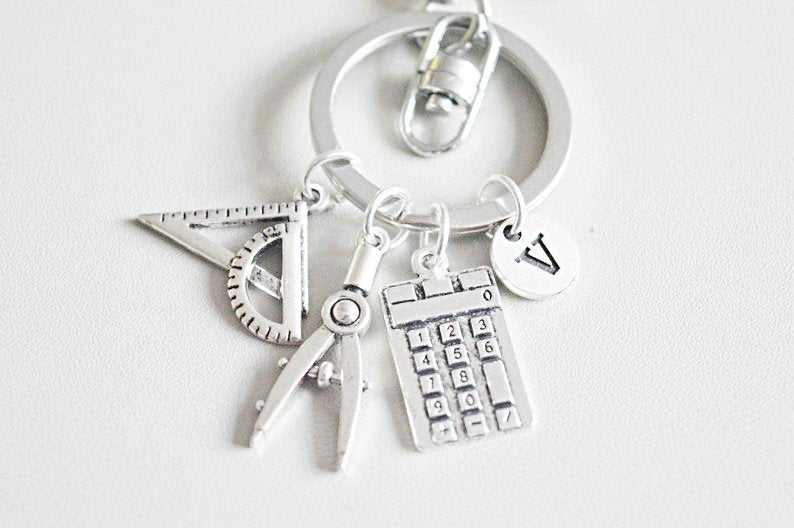# Maths Gift, Maths Gift, Maths Keychain, Maths Keyring, Maths Student, Teacher Maths, Math Teacher, Math Graduation, Maths Class, Mathematics

£9.50

Maths Gift, Maths Gift, Maths Keychain, Maths Keyring, Maths Student, Teacher Maths, Math Teacher, Math Graduation, Maths Class, Mathematics

Add a birthstone for an extra £2

Add initial for an extra £2